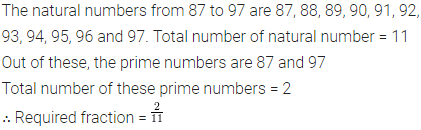# ML Aggarwal Class 6 Solutions for ICSE Maths Chapter 6 Fractions Ex 6.1

## ML Aggarwal Class 6 Solutions for ICSE Maths Chapter 6 Fractions Ex 6.1

Question 1.
Write the following division as fractions:
(i) 3 ÷ 7
(ii) 11 ÷ 78
(iii) 113 ÷ 128
Solution: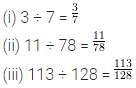Question 2.
Write the following fractions in words.
(i) $$\frac{2}{7}$$
(ii) $$\frac{3}{10}$$
(iii) $$\frac{15}{28}$$
Solution:Question 3.
Write the following fractions in number form:
(i) one – sixth
(ii) three – eleventh,
(iii) seven-fortieth
(iv) thirteen – one hundred twenty fifth
Solution: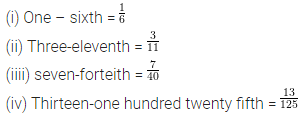Question 4.
What fraction of each of the following is shaded part?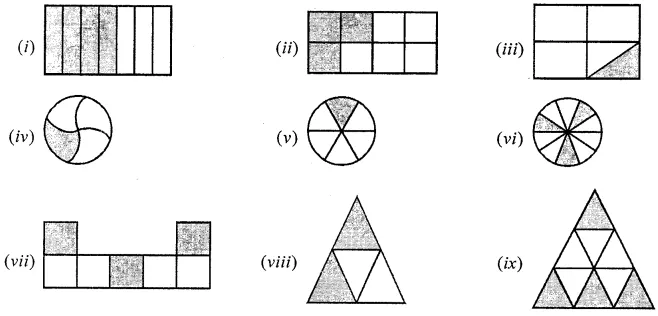Solution: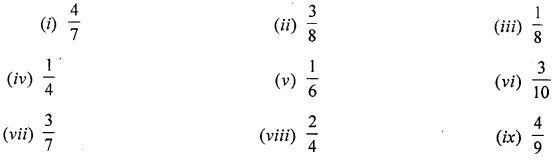Question 5.
Shade the parts of the following figures according to given fractions:Solution: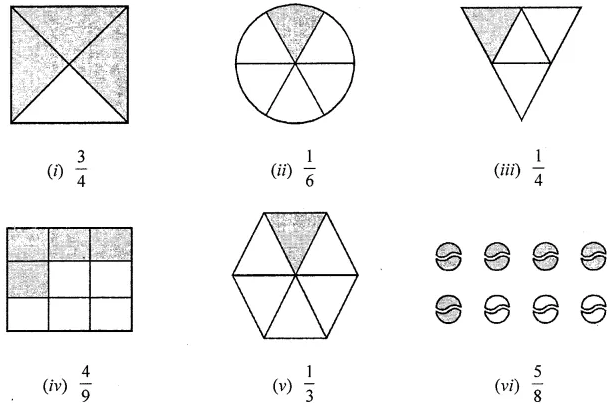Question 6.
In the adjoining figure, if we say that the shaded region is $$\frac{1}{4}$$ of the whole region, then identify the error in it.Solution: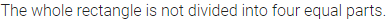Question 7.
Write the fraction in which
(i) numerator = 5 and denominator = 13
(ii) denominator = 23 and numerator = 17
Solution: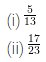Question 8.
Shabana has to stitch 35 dresses. So, ar she has stitched 21 dresses. What fraction of dresses has she stitched?
Solution: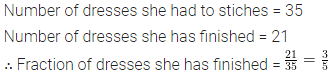Question 9.
What fraction of a day are 8 hours?
Solution:Question 10.
What fraction of an hour are 45 minutes?
Solution:Question 11.
How many natural numbers are there from 87 to 97? What fraction of them are prime numbers?
Solution: